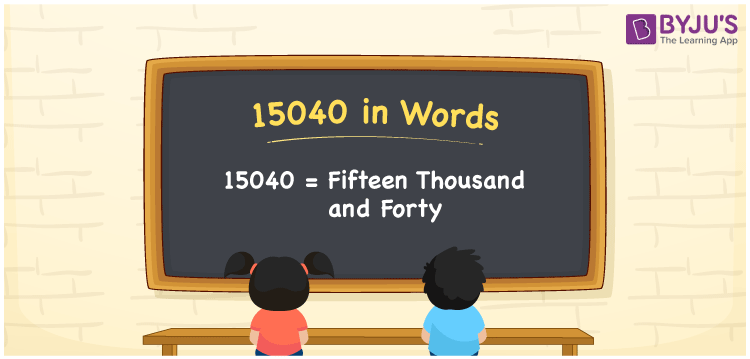# 15040 in Words

We can write 15040 in words as Fifteen thousand and forty. That means the number name of 15040 is Fifteen thousand and forty. Suppose you paid Rs. 15040 for decorating a room; you can say, “I paid Fifteen thousand and forty rupees to decorate a room”. Thus, we use the number name of 15040 to express or spell the number. In this article, you will learn how to derive the cardinal number 15040 into words.

 15040 in words Fifteen thousand and forty Fifteen thousand and forty in Numbers 15040

## 15040 in English Words

Generally, we use the English alphabet to express numbers in words. Thus, we can express 15040 in English words as “Fifteen thousand and forty”.## How to Write 15040 in Words?

Let’s understand how to write the number 15040 in words using a place value chart given below.

 Ten thousand Thousands Hundreds Tens Ones 1 5 0 4 0

Here, ones = 0, tens = 4, hundreds = 0, thousands = 5, ten thousand = 1

These digits can be expanded based on the place values as follows.

1 × Ten thousand + 5 × Thousand + 0 × Hundred + 4 × Ten + 0 × One

= 1 × 10000 + 5 × 1000 + 0 × 100 + 4 × 10 + 0 × 1

= 10000 + 5000 + 40

= Ten Thousand + Five thousand + Forty

= Fifteen thousand + Forty

= Fifteen thousand and forty

Therefore, 15040 in words = Fifteen thousand and forty.

15040 is a natural number that is the successor of 15039 and predecessor of 15041.

15040 in words – Fifteen thousand and forty

Is 15040 an odd number? – No

Is 15040 an even number? – Yes

Is 15040 a prime number? – No

Is 15040 a composite number? – Yes

Is 15040 a perfect square number? – No

Is 15040 a perfect cube number? – No

## Frequently Asked Questions on 15040 in Words

Q1

### How do you write 15040 in words?

We can write 15040 in English words as Fifteen thousand and forty.
Q2

### How do you write an amount of Rs. 15040 in words on a cheque?

On a cheque, we generally write an amount of Rs. 15040 in words as “Fifteen thousand and forty rupees only”.
Q3

### Find 15040 + 460. Write the answer in words.

15040 + 460 = 15500 Thus, the value of 15040 + 460, i.e., 15500 in words is Fifteen thousand five hundred.